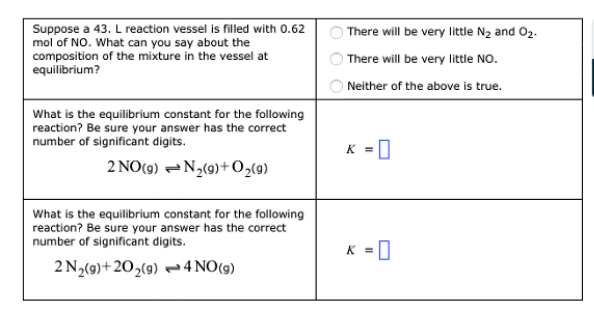Problem: At a certain temperature, the equilibrium constant  K for the following reaction is 225:N2(g) + O2(g) ⇌ 2NO(g) Use this information to complete the following table.

FREE Expert Solution
92% (500 ratings)
FREE Expert Solution
92% (500 ratings)
Problem Details

At a certain temperature, the equilibrium constant  K for the following reaction is 225:

N2(g) + O2(g) ⇌ 2NO(g)

Use this information to complete the following table.What scientific concept do you need to know in order to solve this problem?

Our tutors have indicated that to solve this problem you will need to apply the Chemical Equilibrium concept. You can view video lessons to learn Chemical Equilibrium Or if you need more Chemical Equilibrium practice, you can also practice Chemical Equilibrium practice problems .

What is the difficulty of this problem?

Our tutors rated the difficulty of At a certain temperature, the equilibrium constant  K for th... as medium difficulty.

How long does this problem take to solve?

Our expert Chemistry tutor, Dasha took 5 minutes to solve this problem. You can follow their steps in the video explanation above.

What professor is this problem relevant for?

Based on our data, we think this problem is relevant for Professor Graves' class at FIU.